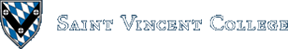Computing & Information Systems    DepartmentSearch CIS Site      Tutorials

## Software Design Using C++

### What is a Queue?

A queue is a "waiting line" type of data structure. Much like with a stack, you can insert items into a queue and remove items from a queue. However, a queue has "first come, first served" behavior in that the first item inserted into the queue is the first one removed. This is sometimes abbreviated FIFO (first in, first out). A queue is also a LILO (last in, last out) data structure. Thus, items are removed from the queue in the very same order in which they were put into the queue in the first place.

### Uses of Queues

Queues are commonly used in operating systems and other software where some sort of waiting line has to be maintained for obtaining access to a resource. For example, an operating system may keep a queue of processes that are waiting to run on the CPU. It might also keep a queue of print jobs that are waiting to be printed on a printer. Queues are also used in simulations of stores and their waiting lines at the check-out counters.

Many of these files are the same as the ones we used in implementing stacks. Then the lstqueue.h file defines the LstQueClass as follows:

 `````` class LstQueClass { public: // No constructor is mentioned here. We instead use the default // constructor automatically supplied by the compiler. bool Empty(void) const; void Insert(const ItemType & Item); void Remove(ItemType & Item); private: ListClass List; // an embedded List object }; ``````

As with a stack, a queue object (a `LstQueClass` object to be precise) contains an embedded list for holding the data. The implementation of the three queue functions is easy, since we can simply call upon the appropriate list-processing functions. To insert an item into a queue, we insert it at the rear of the embedded list. To remove an item from a queue, we remove it from the front of the list. See `lstqueue.cpp` for the details. Then look at `quetest.cpp` for a test program that tries out a queue object.

### A Variant of our Linked List Implementation

Most of these files are the same, but this time we have a `queue.h` file that sets up the following abstract base class:

 `````` class QueBaseClass { public: virtual void Insert(const ItemType & Item) = 0; virtual void Remove(ItemType & Item) = 0; virtual bool Empty(void) const = 0; }; ``````

This tells us that we will have `Insert`, `Remove`, and `Empty` functions. The `lstqueue.h` file then derives the following class by public inheritance from our abstract base class:

 `````` class LstQueClass: public QueBaseClass { public: bool Empty(void) const; void Insert(const ItemType & Item); void Remove(ItemType & Item); private: ListClass List; // an embedded List object }; ``````

### An Array Implementation

It is also possible to implement queues so that a queue object uses an array to hold the data. For example, if we use an array that can hold 5 items, we might have a picture like the following: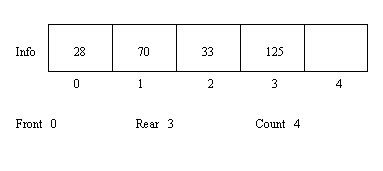This picture shows that the queue contains (in order): 28, 70, 33, 125. Note that 0 for `Front` indicates that the first item in the queue is the 28, the item at index 0. Similarly, the 3 for `Rear` shows that the last item in the queue is the 125, the item at index 3. Of course, 4 for `Count` tells us that there are currently 4 items in the queue.

Let's now remove an item from the queue. Of course, we always remove from the front, so the 28 is removed. The new picture of the queue is as follows: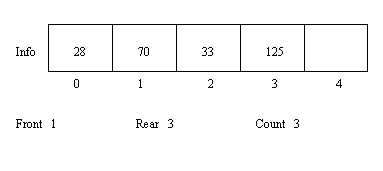Note that no attempt is made to overwrite the 28. The key is to change the `Front` index to show that the queue now runs from index 1 to index 3, that is, the queue contains 70, 33, 125. The 28 is still sitting in there as garbage data.

Next, let's insert 64. This leads to the following picture. Note that we insert at the rear of the queue, of course.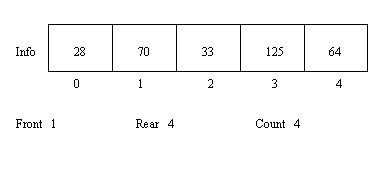Let's next insert 99. You might think that we are out of luck since the last item inserted went at the top index in the array. In such a case you just wrap around to the start of the array and use index 0 if it is unused. This is sometimes referred to as a "circular array", since the effect is the same as what you would get by pasting the two ends of the array together to form a circle. The picture of the queue follows: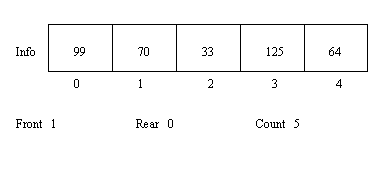Note that the queue is now filled, as you can easily tell by looking at the `Count`. However, we could remove additional items. Once that is done, new items could be inserted. We might wrap around the array many times in the course of using this queue!

A complete example program that uses an array-based queue is given in the following files. The particular test program is essentially the same as the one we used to try out a list-based queue. The first two files are exactly the same as before. In looking at arrqueue.h and arrqueue.cpp you see that we derived the class `ArrQueClass` by public inheritance from the abstract base class `QueBaseClass`. A constructor was added so that the `Front`, `Rear`, and `Count` fields of the newly constructed object are correctly initialized for an empty queue. (To see why `Rear` is initialized as it is, draw a picture of what happens when the first item is inserted into the newly created queue.) A private helping function, called `Advance`, is set up so that we can just call it anytime we want to advance the `Front` or `Rear` to the next index (with wrap around). Note that it is an inline function for efficiency.